# Cellular Automata Example

Outline

• Creating a Cellular Automata Network
• Game of Life
• Patterns
• Cellular Automata on a Torus

Mango can be used to visualize Cellular Automata where each cell is a node and neighbors are linked together. This is an example using scripts and patterns at the following github page:

https://github.com/j23414/Visual_CA.git

In cellular automata, a cell is in one of a finite set of states (often either alive, or dead). At each epoch, or time step, the next state is determined the by the current state of its immediate neighbors.

Often you are defining an initial pattern and then run it for a few steps to notice if there is a loop or some complexity arising from a simple set of rules.

## Creating a Cellular Automata Network

For example, there is the blinker pattern where 0 represent dead and 1 represents alive:

0,0,0,0,0
0,0,0,0,0
0,1,1,1,0
0,0,0,0,0
0,0,0,0,0


I have provided a perl script to convert this pattern to a Mango readable network:

#! /usr/bin/perl

use strict;
use warnings;

my $id=0; my$x=0;
my $y=0; my @nodes; while(<>){ chomp; @nodes = split(/,/);$x=0;
foreach my $state (@nodes){ print "n".($id++).",$state,".($x++).",".($y*-1)."\n"; }$y++;
}
print "-\n";
my $i; my$j;
for($i=0;$i<$y;$i++){
for($j=1;$j<$x;$j++){
print "n".($i*$x+$j-1).",n".($i*$x+$j)."\n"; # -
if(($i*$x+$j-1)-($x)>=0){
print "n".($i*$x+$j-1-$x).",n".($i*$x+$j-1)."\n"; # | print "n".($i*$x+$j-$x).",n".($i*$x+$j-1)."\n";   # /
if($j>0){ print "n".($i*$x+$j-1-$x).",n".($i*$x+$j)."\n"; # \
}
}
}
if(($i*$x+$j-1)-($x)>=0){
print "n".($i*$x+$j-1-$x).",n".($i*$x+$j-1)."\n"; # | last } } for(my$i=0; $i<$x; $i++){ for(my$j=1; $j<$y; $j++){ print "n".($i*$y+$j-1).",n".($i*$y+$j)."\n"; } }  Run the following to generate the network file $ perl pattern2net.pl blinker.txt > blinker.net


Within Mango, navigate to the folder containing the files you cloned from the github site. Open the gel.txt file and run the top commands to load blinker.net and set default layout:

/* load cellular automata pattern */
node(string name, int state, float x, float y, int delta) nt;

/* layout */
foreach node in ca set _x=x, _y=y, _g=0, _b=0;
center(ca);
foreach node in ca where state==1 set _r=1;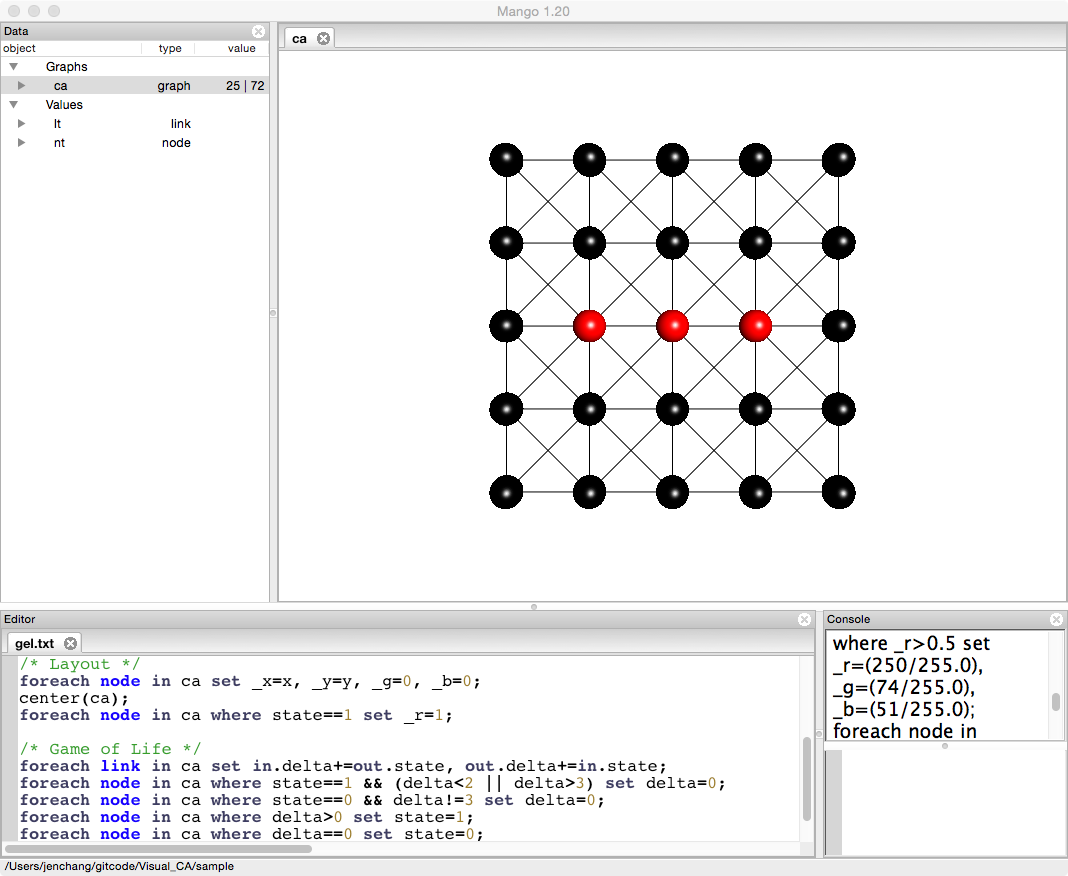## Game of Life

To run one step, select and run the following lines:

/* Game of Life */
foreach link in ca set in.delta+=out.state, out.delta+=in.state;
foreach node in ca where state==1 && (delta<2 || delta>3) set delta=0;
foreach node in ca where state==0 && delta!=3 set delta=0;
foreach node in ca where delta>0 set state=1;
foreach node in ca where delta==0 set state=0;
foreach node in ca set _r=state, delta=0;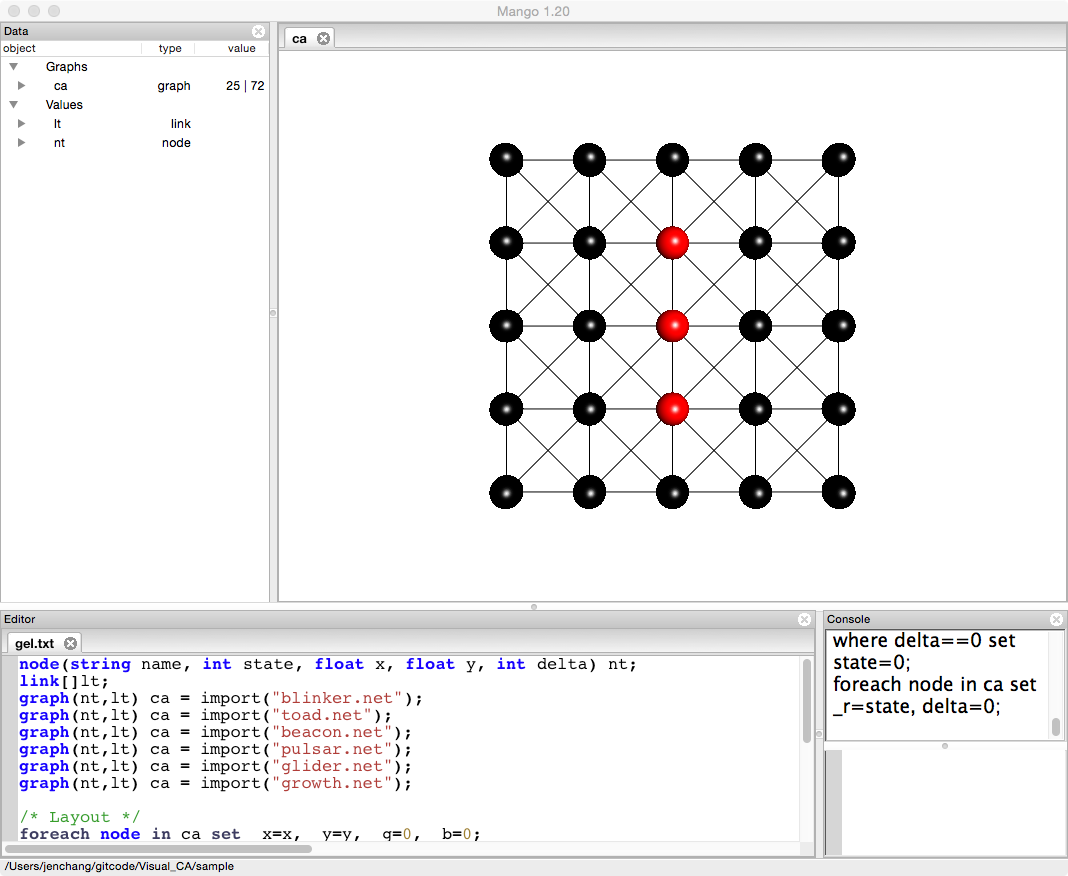Rerun that comand to continue to blink back and forth between the patterns. Other patterns such as toad, beacon, pulsar, glider, growth are provided.

## Patterns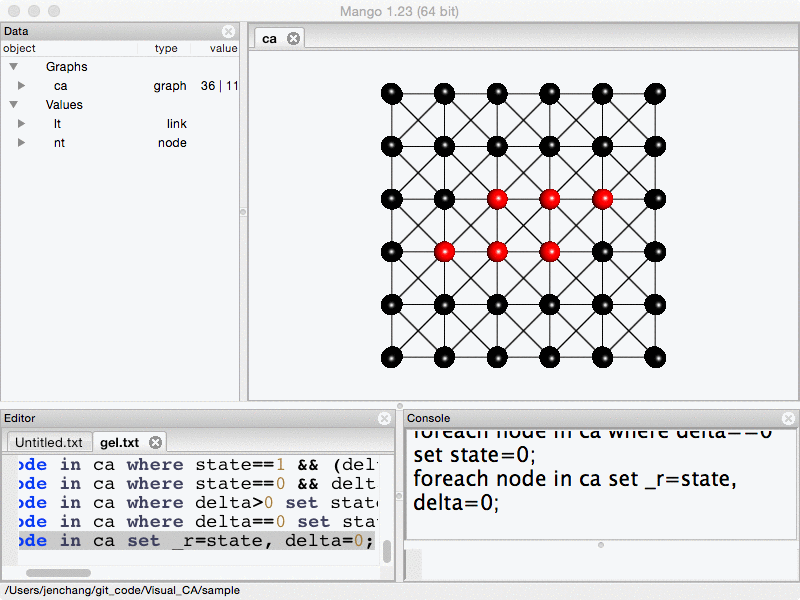### beacon.net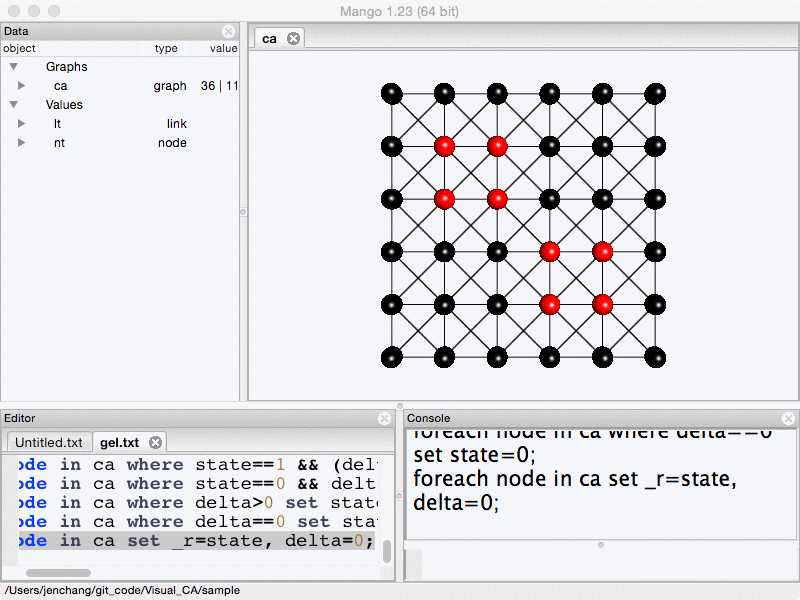### pulsar.net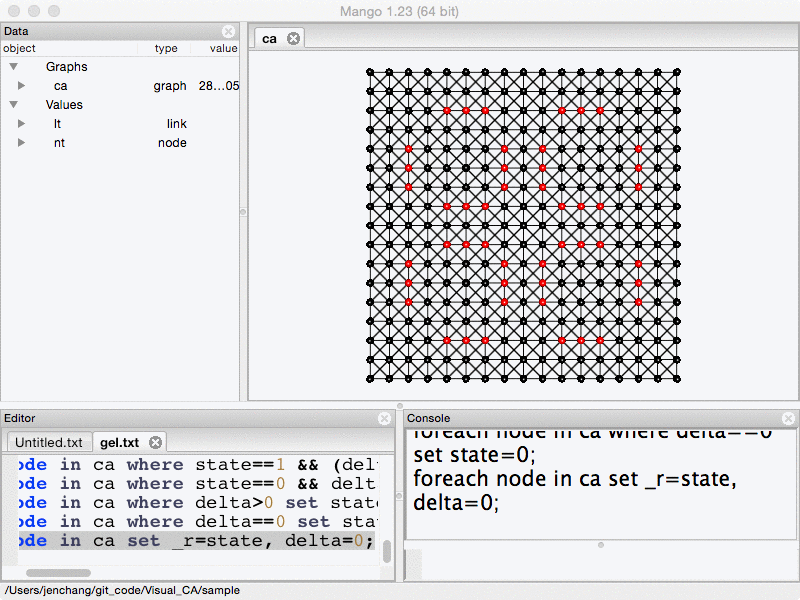## Cellular Automata on a Torus

Perl script converting pattern to a torus network

#! /usr/bin/perl

use strict;
use warnings;

my $usage = << 'USAGE'; Usage:$0 [width height] pattern.txt
width: cellular automata width (default 40)
height: cellular automata height (default 40)
pattern.txt: cellular automata patterns to be loaded
The dimension options, if given, must be both provided
USAGE

my ($width,$height) = (40, 40);
if (@ARGV==3) {
$width = shift @ARGV;$height = shift @ARGV;
} elsif (@ARGV!=1) {
die $usage; } my$pi = 3.1415926535897932384626433;
my $size = 5.0; my$scale = 2.5;
my $map_x =$width/4;
my $map_y =$height/4;

my @torus;
for (my $j=0;$j<$height; ++$j) {
for (my $i=0;$i<$width; ++$i) {
my $circle = 2.0*$pi;
my $y = cos($j*$circle/$height)*$scale; my$diameter = $size+2*sin($j*$circle/$height);
my $x =$diameter/2*cos($i*$circle/$width)*$scale;
my $z =$diameter/2*sin($i*$circle/$width)*$scale;
push @{$torus[$j][$i]}, "n_${i}_${j}",$x, $y,$z, 0;
}
}
for (my $j=$map_y; <>; ++$j){ chomp; my @nodes = split(/,/); for (my$i=0; $i<@nodes; ++$i) {
$torus[$j%$height][($map_x+$i)%$width] = $nodes[$i];
}
}

for (@torus) {
for (@{$_}) { print join(",", @{$_}), "\n";
}
}
print "-\n";

for (my $j=0;$j<$height; ++$j) {
for (my $i=0;$i<$width; ++$i) {
print $torus[$j][$i],",",$torus[$j][($i+1)%$width],"\n"; print$torus[$j][$i],",",$torus[($j+1)%$height][($i+1)%$width],"\n"; print$torus[$j][$i],",",$torus[($j+1)%$height][$i],"\n";
print $torus[$j][$i],",",$torus[($j+1)%$height][($i-1)%$width],"\n";
}
}


Command line

\$ perl pattern2torus.pl glider.txt > glider.net


Gel Script

/* load cellular automata pattern */
node(string name, float x, float y, float z, int state, int delta) nt;
graph(nt,lt) ca = import("glider.net");

/* layout */
foreach node in ca set _x=x, _y=y, _z=z, _r=state, _g=_b=0.50;
center(ca);

/* you may jump below to run the Game of Life rules to see how the
glider moves across ca space, or you can randomize the ca graph
and see how John Conway sees it but now in 3D graph!! */
foreach node in ca set state = rand(0,1);

/* Game of Life rules */
foreach link in ca set in.delta+=out.state, out.delta+=in.state; foreach node in ca set state=state==1&&(delta<2||delta>3) ? 0 : state==0&&delta==3 ? 1 : state, _r=state, delta=0;